Study Guide

# Geometric Probability

## Geometric Probability

If we’re taking a nap on a blanket at the park, and a bird poops in the park, what are the chances of the blanket getting hit by the poop? Believe it or not, we can figure that out using probability – geometric probability. In probability, we divide how many desired outcomes there are by how many total outcomes there are. In geometric probability we divide the desired area (desired by the bird that is) by the total area.

Let’s say the park is 50 feet wide and 100 feet long, and we’re sitting on a blanket that is 5 ft wide and 10 ft long.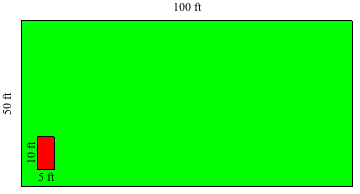We can divide the area of the blanket (aka the bird’s target) by the area of the whole park to get the geometric probability. In this case, it’d be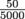or 0.01. Then, we can move the decimal two places to the right to convert to a percent, and we get 1%. Looks like we’re safe hanging out there in the park for a while.

What if we bring a blanket that is 40 ft wide and 60 ft long to the same park? First, we’d need a tractor to help us spread it out. Second, the geometric probability of getting bird poop on this blanket increases, a lot. Now the desired area is 40 × 60 = 2400 ft2. The geometric probability has increased to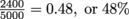.

Let’s look at a different scenario. Say the bird was in a wire cage directly above our blanket, positioned so the total area that the bird’s poop can land on is covered by our blanket. What’s the geometric probability that its poop will land on the blanket? The total area is now the exact same as the target area, so the geometric probability is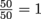meaning there’s a 100% chance it will land on the blanket.

Luckily, a Parks and Rec employee moves the caged bird so it isn’t anywhere near our blanket. What are the chances of getting pooped on by the bird now? Because the target area does not include the blanket, the chances are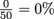. Much better odds for a nap in the park.